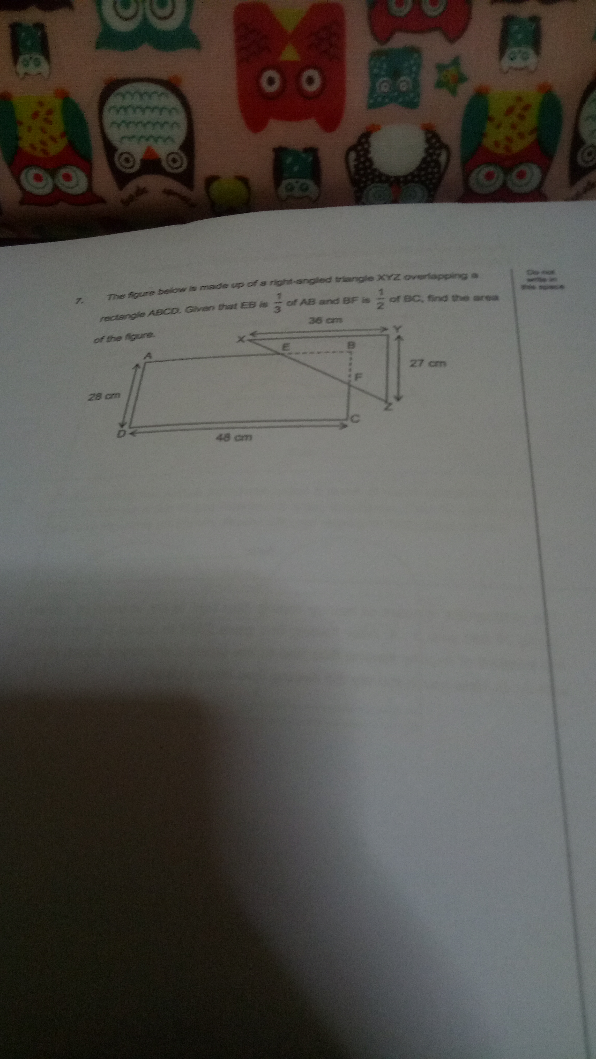# QuestionCan’t see the picture clearly…hope I got the numbers correct.

EB = 1/3 × 48 = 16 cm

BF = ½ × 28 = 14 cm

Area of ΔBEF = ½ × 16 × 14 = 112 cm²

Area of rectangle = 48 × 28 = 1344 cm²

Area of ΔXYZ = ½ × 36 × 27 = 486 cm²

Area of figure -> 1344 + 486 – 112 = 1718 cm²

0 Replies 1 Like ✔Accepted Answer